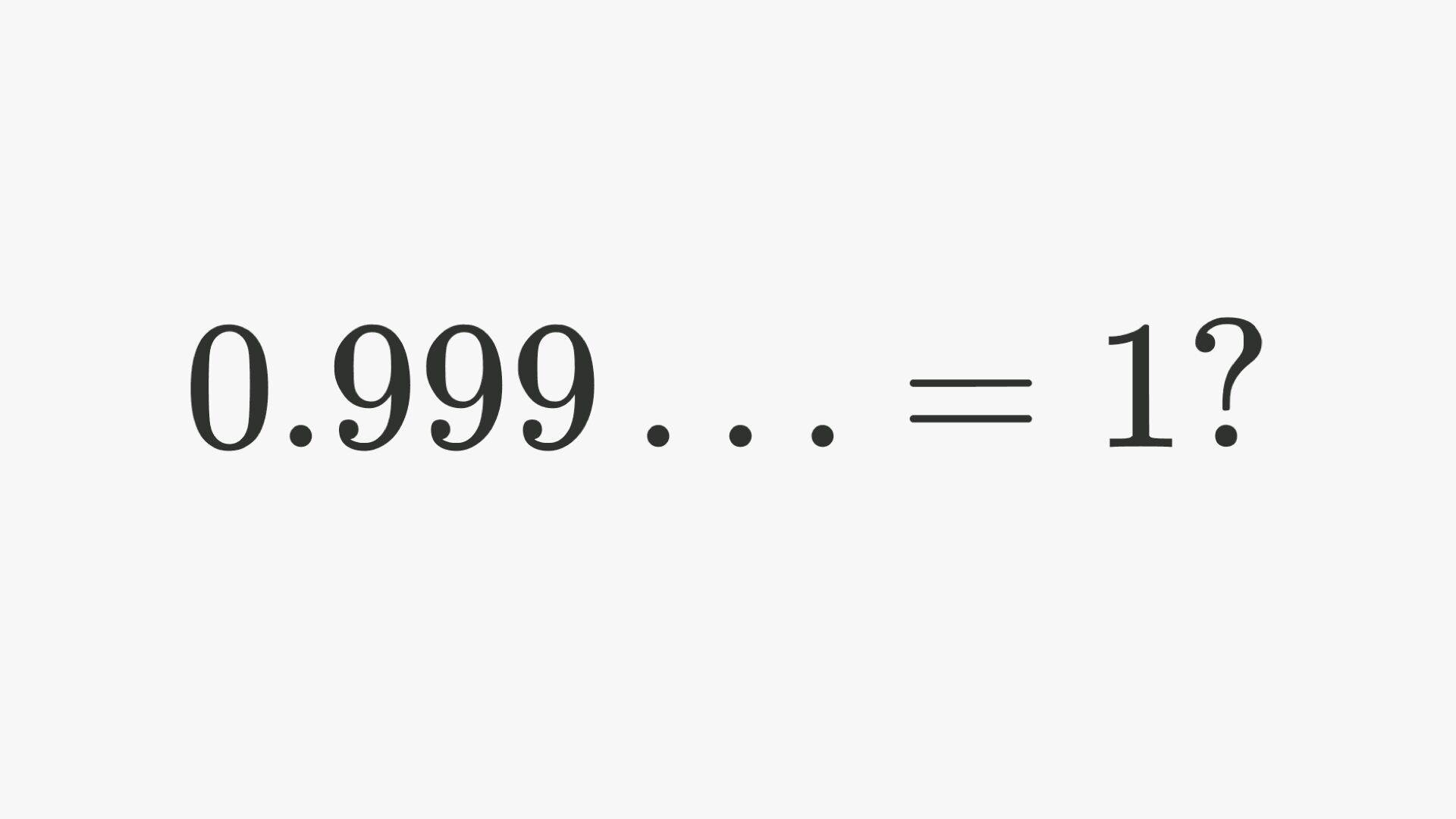# Yes Virgina .999… really does equal 1.I have recently been inundated by number of people who for some strange reason can not seem to accept the mathematical fact that .999… = 1. Not just an approximation, not just math tricks, but they are in fact *exactly* equal just as 1/2 = .5 is a objective mathematical fact. There are many things like life which are debatable…mathematical facts are simply not one of them.

If you ever find anyone thinks otherwise, challenge them to answer these 4 questions I wrote:

1) ALL rational numbers by definition can be expressed in the form of a ratio of two integers p/q as ℚ = {p/q | (p,q)=1, p,q ∈ ℤ where q ≠ 0}. Since .999… is rational by definition as all repeating decimals are rational. It has to be able to be expressed in the form p/q which is the ratio of two integers. If .999… is rational by definition what is p/q = .999…?

2) .999… = lim k→∞ Σ 9/10^n, n=1 to k by the definition of a limit of a sequence.  lim k→∞ Σ 9/10^n, n=1 to k = 1. If both .999… and 1 = lim k→∞ Σ 9/10^n, n=1 to k please explain how they are not equal.

3) 1- .999… = x. What is x? infinitesimally small values are not allowed in ℝ due to the Archimedean property so given no infinitesimals can exist in ℝ please give the real value of x if x ≠ 0. (If you claim x = 0.000…1 then please construct 0.000…1 using the definition of a Cauchy sequence ε > 0 there exists N such that if m, n > N then |am- an| < ε and please give the sequence and show it is convergent upon 0.000…1).

4) By the trichotomy property you can only have x<y, x=y, x>y. If x <> y then there exists (x+y)/2 between them else as the reals are dense else x=y. If x=1 and y=.999… what is (x+y)/2?

Yes Virginia, using REAL math 1=.999… is absolutely true.

1..999… is not exactly equal to 1 because it is strictly less than 1.

Consider 2 minus .999… It is strictly greater than 1. If .999… is exactly equal to 1 then .999… and 2 minus .999… are both equal to 1 but they can’t be equal if one is strictly less than 1 and the other is strictly greater than 1.

2.That was a fun read, Steve.

Here’s one more way to think about it.

Let,
x = 0.9999…

Then,
10x = 9.9999…
10x − x = 9.9999… − 0.9999…
9x = 9
x = 1

So, , . . 0.9999… = 1

-Suj

This site uses Akismet to reduce spam. Learn how your comment data is processed.